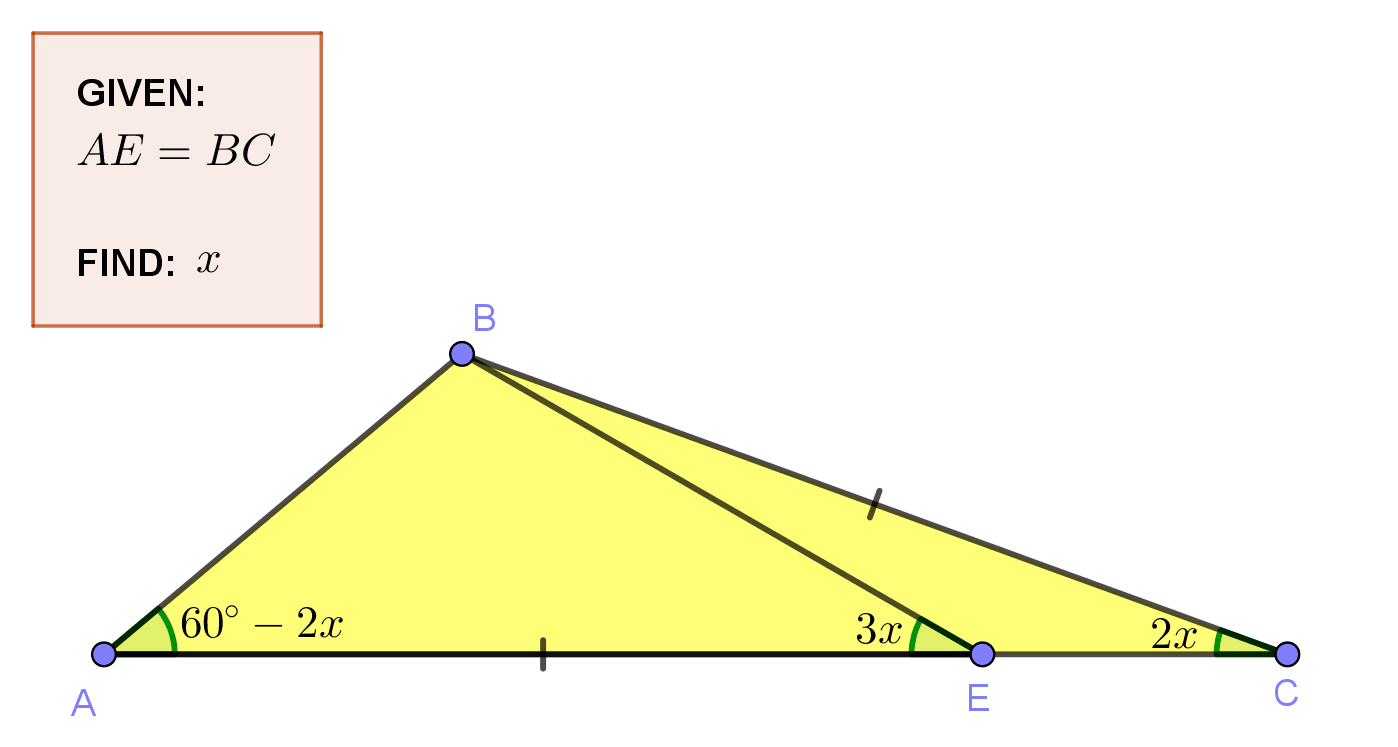# Geometry Problem 1499: Triangle Angles, Cevian, Congruence, Isosceles and Equilateral Triangles, and Auxiliary Construction

In triangle ABC, point E lies on $${\overline {AC}}$$ such that $${\angle{BCA}}={2x},{\angle{BEA}}={3x},{\angle{A}}={60^\circ-2x}$$ and AE = BC. Find the value of $${x}$$.
Auxiliary construction is an extra line, point or circle needed to complete a proof or solution in geometry.## Typography and Sketch for Problem 1498: Chichen Itza in the Background### Geometric Art using Mobile Apps

Geometric figures, which are any set or combination of points, lines, surfaces, and solids, form the basis of geometric art. With the rise of digital art, many artists now use mobile apps to create stunning works of art using these figures.